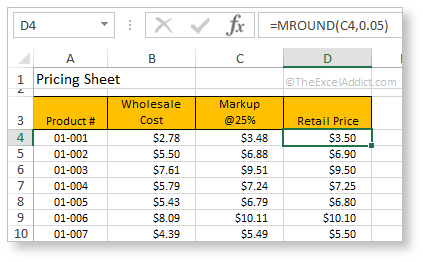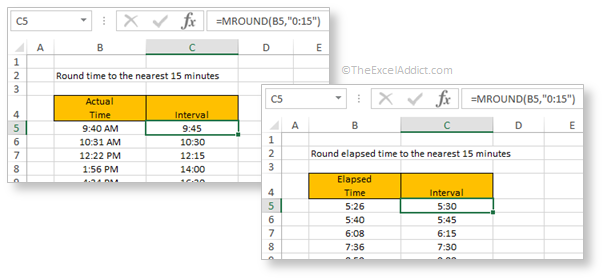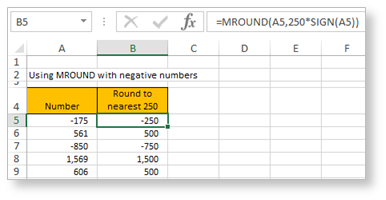# Round Numbers To The Nearest MultipleWhen you need to round a number to a specified multiple, such as rounding a price to the nearest 5¢ or rounding a time to the nearest 15 minutes, Excel’s MROUND is the answer.

For example, several years ago in Canada our 1 cent coin was discontinued, so most cash transactions are now rounded to the nearest 5 cents.

Syntax for the MROUND Function:
=MROUND(number,multiple)

There are two arguments in the MROUND function. number is the number that you want to round. multiple is the multiple to use when rounding.

The MROUND function rounds a number to the nearest multiple of the second argument. For example, in the above example, all retail prices have to be rounded to the nearest 5¢. To calculate the retail prices for all products we use this MROUND formula…
=MROUND(C4,0.05)

Using MROUND for Time
You can also use the MROUND function to round a time to a specified increment. If you want to round a time to the nearest 15 minutes, use the formula…
=MROUND(B5,”0:15″)Using MROUND with Negative numbers
Keep in mind that both arguments in the MROUND function must have the same sign. Therefore, if cells referenced by MROUND have a mix of positive and negative amounts, you will need to multiply the second argument by the sign of the first argument using the SIGN function.

In the example below, =MROUND(A5,0.25*SIGN(A5)) will work for both positive and negative values. The SIGN function returns a 1 if the sign is positive and -1 if the sign is negative.